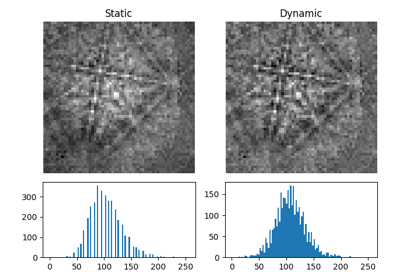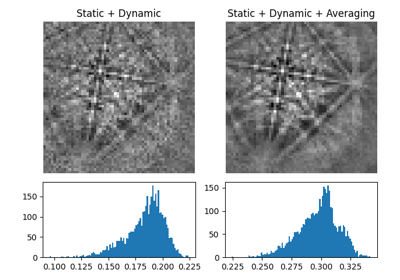# remove_dynamic_background#

EBSD.remove_dynamic_background(operation: str = 'subtract', filter_domain: str = 'frequency', std: = None, truncate: = 4.0, show_progressbar: = None, inplace: bool = True, lazy_output: = None, **kwargs) [source]#

Remove the dynamic background.

The removal is performed by subtracting or dividing by a Gaussian blurred version of each pattern. Resulting pattern intensities are rescaled to fill the input patterns’ data type range individually.

Parameters:
operation

Whether to `"subtract"` (default) or `"divide"` by the dynamic background pattern.

filter_domain

Whether to obtain the dynamic background by applying a Gaussian convolution filter in the `"frequency"` (default) or `"spatial"` domain.

std

Standard deviation of the Gaussian window. If None (default), it is set to width/8.

truncate

Truncate the Gaussian window at this many standard deviations. Default is `4.0`.

show_progressbar

Whether to show a progressbar. If not given, the value of `hyperspy.api.preferences.General.show_progressbar` is used.

inplace

Whether to operate on the current signal or return a new one. Default is `True`.

lazy_output

Whether the returned signal is lazy. If not given this follows from the current signal. Can only be `True` if `inplace=False`.

**kwargs

Keyword arguments passed to the Gaussian blurring function determined from `filter_domain`.

Returns:
`s_out`

Background corrected signal, returned if `inplace=False`. Whether it is lazy is determined from `lazy_output`.

Examples

Remove the static and dynamic background

```>>> import kikuchipy as kp
>>> s = kp.data.nickel_ebsd_small()
>>> s.remove_static_background()
>>> s.remove_dynamic_background(operation="divide", std=5)
```

## Examples using `EBSD.remove_dynamic_background`#Dynamic background correction

Dynamic background correctionNeighbour pattern averaging

Neighbour pattern averaging